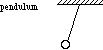index: click on a letter A B C D E F G H I J K L M N O P Q R S T U V W X Y Z A to Z index index: subject areas numbers & symbols sets, logic, proofs geometry algebra trigonometry advanced algebra & pre-calculus calculus advanced topics probability & statistics real world applications multimedia entrieswww.mathwords.com about mathwords website feedback

 Period of Periodic Motion The time needed to complete a cycle. For example, a pendulum exhibits periodic motion. Its period is the time it takes for the pendulum to swing from one side to the other and then back again. Note: Period is the reciprocal of frequency.See also